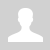Python. PyGame. Keyboard events

12.5.3.4 control characters with the keyboard.

Python. PyGame. Keyboard events

Pygame has a keyboard module. It allows you to get information about pressing buttons on the keyboard. We call them events.
Each event is a user action. For example, the player controls the object using the keyboard buttons to move the object up, down, left, right, etc.
At each stage of the main game loop, events are programmed to control the game.
Despite the fact that the loop is high-speed, there can be several events in one iteration. Therefore, a second inner loop appears in the program, which processes all the events that have occurred (breaks the sequence of events).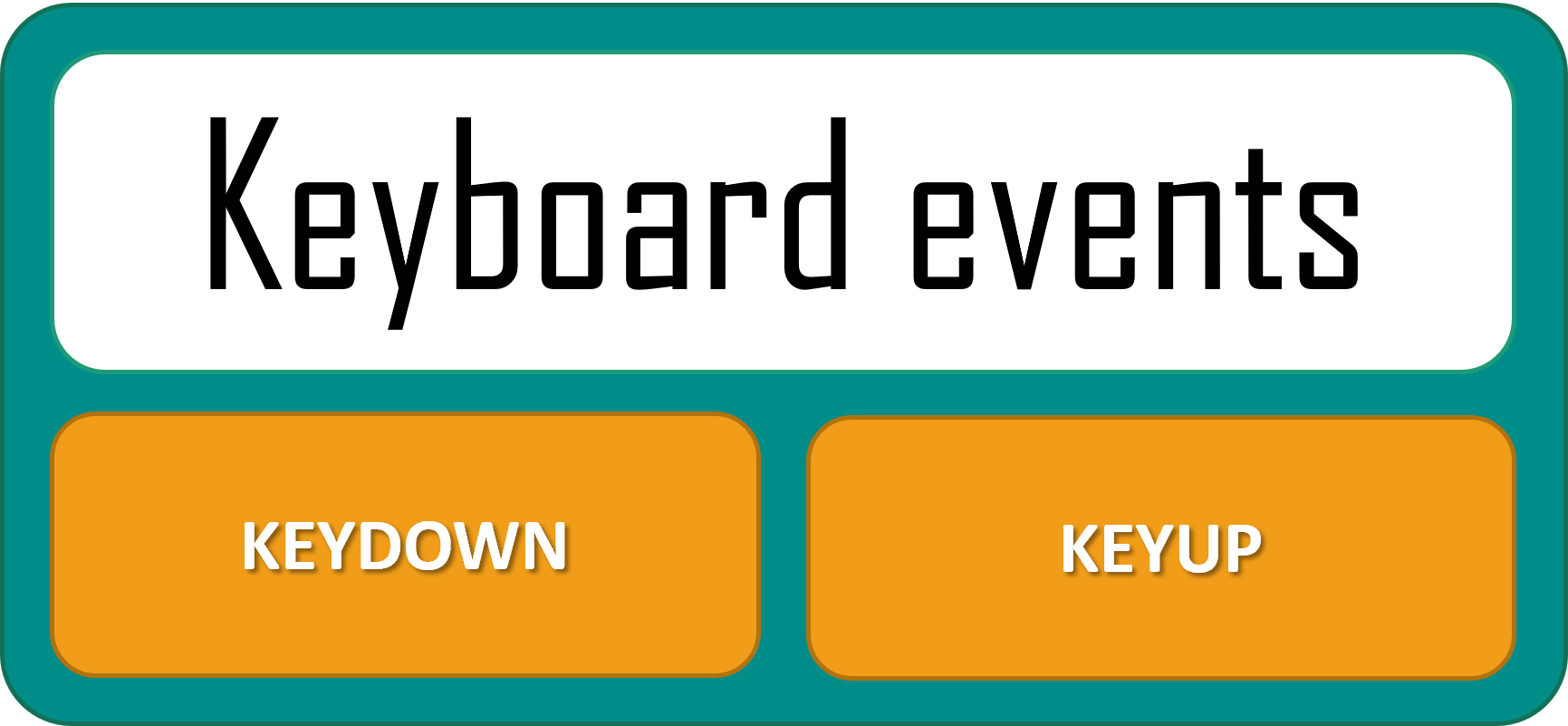Pygame handles two types of keyboard events:

• Press the key (the value of the type property of the event corresponds to the pygame.KEYDOWN constant).
• Key release (pygame.KEYUP).

Keyboard events attribute

event.type attributes
KEYDOWN key, mod (for example, CTRL, SHIFT, etc), unicode
KEYUP key, mod

Check keydown

pygame.key.get_pressed() – a method that gets the state of all keyboard buttons, i.e. with this method we can check if any key has been pressed.
To check if a key has been pressed use the following code:

```if event.type == pygame.KEYDOWN:     if pygame.key.get_pressed():         print("You are pressed any key")```

The key is used to check if a particular key has been pressed. You can define a key in the following way:

For example, pygame.K_LEFT

 Key Keyboard button K_UP up arrow K_DOWN arrow to down K_LEFT left arrow K_RIGHT right arrow

Other codes of keys

Task 1. Write a program in which a circle with a radius of 20 increases by 20 pixels each time a keyboard button is pressed.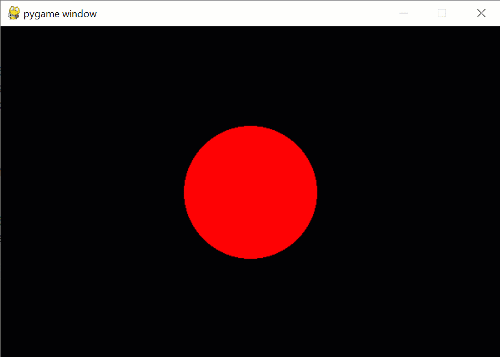Decision

import pygame

size = width, height = 600, 400
screen = pygame.display.set_mode(size)
clock = pygame.time.Clock()
x0 = width // 2
y0 = height // 2
R = 20
pygame.draw.circle(screen, (255, 0, 0), (x0, y0), R)
running=True
while running:
#screen.fill((0, 0, 0))
for event in pygame.event.get():
if event.type == pygame.QUIT:
running = False
if event.type == pygame.KEYDOWN:
if pygame.key.get_pressed():
screen.fill((0, 0, 0))
R += 10
pygame.draw.circle(screen, (255, 0, 0), (x0, y0), R)
clock.tick(50)
pygame.display.flip()
pygame.quit()

Task 2. Write a program in which a circle with a radius of 100 moves the circle 5 pixels to the right each time the "right" key is pressed, and each time the "left" key is pressed, it moves the circle 5 pixels to the left.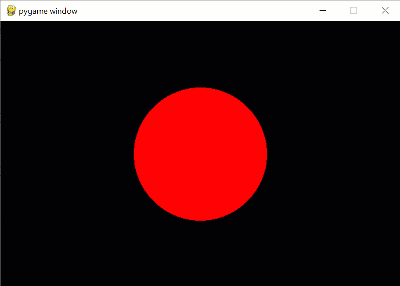Decision

import pygame

size = width, height = 600, 400
screen = pygame.display.set_mode(size)
clock = pygame.time.Clock()
x0 = width // 2
y0 = height // 2
R = 100
pygame.draw.circle(screen, (255, 0, 0), (x0, y0), R)
running=True
while running:
#screen.fill((0, 0, 0))
for event in pygame.event.get():
if event.type == pygame.QUIT:
running = False
if event.type == pygame.KEYDOWN:
if event.key == pygame.K_RIGHT:

x0 += 5
if event.key == pygame.K_LEFT:
x0 -= 5
screen.fill((0, 0, 0))
pygame.draw.circle(screen, (255, 0, 0), (x0, y0), R)

clock.tick(50)
pygame.display.flip()
pygame.quit()

But it is not the most efficient solution, since in the game to move to the right, you need to constantly press and release the key, so we will move the keypress check to the main game loop.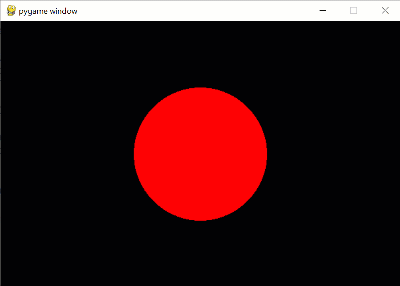Decision

import pygame

size = width, height = 600, 400
screen = pygame.display.set_mode(size)
clock = pygame.time.Clock()
x0 = width // 2
y0 = height // 2
R = 100
pygame.draw.circle(screen, (255, 0, 0), (x0, y0), R)
running=True
while running:
#screen.fill((0, 0, 0))
for event in pygame.event.get():
if event.type == pygame.QUIT:
running = False
keys = pygame.key.get_pressed() # get the state of all keys
if keys[pygame.K_LEFT]: # if pressed key right
x0 -= 2
elif keys[pygame.K_RIGHT]: # if pressed key left
x0 += 2
screen.fill((0, 0, 0))
pygame.draw.circle(screen, (255, 0, 0), (x0, y0), R)

clock.tick(50)
pygame.display.flip()
pygame.quit()

Using keyboard shortcuts

Information about the modifier is contained in the mod attribute of the pygame.KEYDOWN and pygame.KEYUP events. The mod attribute is a bitmask of all modifier keys that were pressed when the event occurred. The modifier information can be decoded using a bitwise AND.

For example,

```if event.type == pygame.KEYDOWN:     if event.key == pygame.K_s and (event.mod & pygame.KMOD_CTRL):         print("Ctrl + 'S' was pressed!") # Perform the shortcut operation         pygame.key.set_mods(0) # Disable the Ctrl key```

 pygame Constant Description KMOD_LSHIFT left shift KMOD_RSHIFT right shift KMOD_SHIFT left shift or right shift or both KMOD_LCTRL left control KMOD_RCTRL right control KMOD_CTRL left control or right control or both KMOD_LALT left alt KMOD_RALT right alt KMOD_ALT left alt or right alt or both KMOD_LMETA left meta KMOD_RMETA right meta KMOD_META left meta or right meta or both KMOD_CAPS caps lock KMOD_NUM num lock KMOD_MODE AltGr

Questions:

1) Name the keyboard events when you can program it in PyGame.

2) Explain how to organize movement according to the keyboard.

3) How can we control several object movements at the same time?

Exercises: